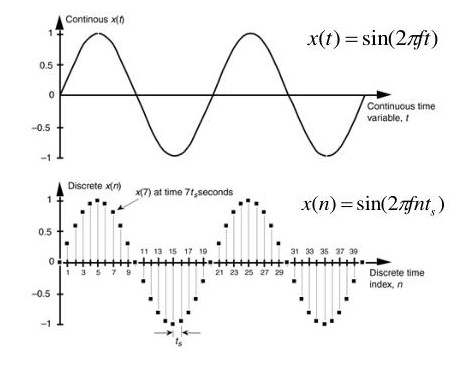This tutorial will discuss using analog pins to read an analog signal and convert it into digital in Arduino.

The ADC, also known as analog to digital converter, converts an analog signal to a digital signal. Analog signals are continuous, which means they have an infinite number of values inside them. We cannot read all the values, so we read values at specific intervals of time from the continuous signal, making the signal digital.

The process of taking samples from the analog signal is called sampling. The process of converting an analog signal to digital is shown in the below diagram.The first signal in the above diagram is a continuous signal with infinite values, and the second signal is a digital signal with a finite value. The analog to digital converter takes values at regular intervals from the analog signal to make it a digital signal.

Analog to digital conversion is done because a computer cannot process an analog signal; it can only process digital signals in 0s and 1s. After processing the digital signal, we can also convert the signal back to analog using DAC or digital to analog converter.

For example, joining the points in the second signal in the above diagram will become almost identical to the first signal. The number of samples we take in the ADC is called the ADC’s sampling rate or bit rate.

Some Arduino boards have 10-bit analog to digital converters, and some have a 12-bit analog to digital converters. The bit rate is also called the resolution, and it defines the number of samples the ADC will take from the analog signal.

A 10-bit analog to digital converter will take 1024 samples from the analog signal. For example, suppose the input signal consists of voltage levels between 0 to 5 volts.

In that case, the voltage levels will be mapped to the integer range of 0 to 1024, which means the analog to digital converter will take a sample after every 51024 or 4.9 millivolts.

The speed of the analog to digital converter depends on the operating frequency of the Arduino board in `ATmega` based Arduino boards; the analog to digital converter will take 100 microseconds to convert an analog signal to a digital signal which means the sampling rate or reading rate is 10 kHz or 10,000 times a second.

Other boards like the Arduino Zero, Due, and MKR family boards have 12-bit analog to digital converters, which means the input analog signal of 0 to 3.3 volts will be mapped to an integer range of 0 to 4095.

By default, the 12-bit analog to digital converters will be set to 10-bits, but we can change the bit rate or resolution of the analog to digital converter using the `analogReadResolution()` function, passing the number of bits inside the function to set the resolution.

Check this link for more details about the `analogReadResolution()` function.

The analog pins on every Arduino board are labeled with `A` followed by a number like `A0`, `A1`, etc. To read a signal from an analog pin of Arduino, we can use the `analogRead()` function.

The basic syntax of the `analogRead()` function is below.

``````output = analogRead(pin)
``````

The above syntax will return the analog signal from the analog pin as a digital signal. If the analog pin is not connected to any signal, the function will still return some floating values.

Different Arduino boards have different speeds for the analog to digital converter, which can be changed, but it will also change the resolution of the analog to digital converter. So, we should use the default settings for the analog to digital converter for an accurate analog to digital conversion.

We discussed above that some Arduino boards have 0 to 5 volts reference voltage for the input analog signals, and some have 0 to 3.3 volts. These are the default values, and we can change them using the `analogReference()` function.

We can pass different options depending on different types of Arduino boards.

For example, in the case of Arduino Uno and Mega, we have options like:

1. `DEFAULT` - set the reference voltage to the integer range of 0 to 5 volts.
2. `INTERNAL` - set the reference voltage to the integer range of 0 to 1.1 volts.
3. `INTERNAL1V1` - set the reference voltage to the integer range of 0 to 1.1 volts.
4. `INTERNAL2V2.56` - set the reference voltage to the integer range of 0 to 2.56 volts.

Before setting the reference voltage, note that we cannot set a voltage value below 0 volts and more than 5 volts; the reference voltage value should be 0 to 5 volts.

Suppose we connect a resistor to the analog pin. In that case, the input voltages will be reduced because there is already a resistor of `32K` value present inside the analog pin, and in this case, both resistors will make a voltage divider, and the input voltage will be reduced depending on the value of the resistor.

Check this link for a full list of options that we can use inside the `analogReference()` function. Check this link for more details about the `analogRead()` function.